# Texas Go Math Grade 3 Lesson 7.2 Answer Key Multiply with 5 and 10

Refer to our Texas Go Math Grade 3 Answer Key Pdf to score good marks in the exams. Test yourself by practicing the problems from Texas Go Math Grade 3 Lesson 7.2 Answer Key Multiply with 5 and 10.

## Texas Go Math Grade 3 Lesson 7.2 Answer Key Multiply with 5 and 10

Essential Question
How can you multiply with 5 and 10?

Unlock the Problem
Marcel is making 6 doll-sized banjos. He needs 5 strings for each banjo. How many strings does he need?

• How many banjos is Marcel making?
6 doll-sized banjos is Marcel making.
Explanation:
Number of banjos is Marcel making = 6.

• How many strings does each banjo need?
5 strings for each banjo needs.

Explanation:
Number of 5 strings for each banjo needs = 5.

Use skip counting.
Skip count by 5s until you say 6 numbers.
5, __, ___, ___, ___, ____
6 × 5 = ___
So, Marcel needs ___ strings.
So, Marcel needs 30 strings.

Explanation:
Skip count by 5s until you say 6 numbers.
5, 10, 15, 20, 25, 30.
6 × 5 = 30.

Example
Use a number line.
Each string is 10 inches long. How many inches of string will Marcel use for each banjo?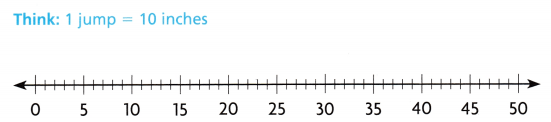• Draw 5 jumps for the 5 strings. Jump 10 spaces at a time for the length of each string.
• You land on 10, __, ___, __, and ___. 5 × 10 = ___

The numbers 10, 20, 30, 40, and 50 are multiples of 10.
So, Marcel will use __ inches of string for each banjo.

A multiple of 10 is any product that has 10 as one of its factors.

Math Talk
Mathematical Processes
What do you notice about the multiples of 10?
Its noticed that multiples of 10 are having zeros in ones place.

Explanation:
Noticed that multiples of 10 are having zeros in ones place.

Share and Show
Question 1.
How can you use this number line to find 8 × 5?Explanation:
Number line is used as:
8 × 5  means multiplying 5 eight times.
=> 8 × 5 = 40.

Math Talk
Mathematical Processes
Explain how knowing 4 × 5 help you find 4 × 10.
4 × 10 = (4 × 5) + (4 × 5) = 40.

Explanation:
4 × 10 = (4 × 5) + (4 × 5)
= 20 + 20
= 40.

Find the product.
Question 2.
2 × 5 = ___
2 × 5 =10.

Explanation:
2 × 5 = (1 × 5) + (1 × 5)
= 5 + 5
= 10.

Question 3.
__ = 6 × 10
60 = 6 × 10.

Explanation:
6 × 10 = (6 × 5) + (6 × 5)
= 30 + 30
= 60.

Question 4.
___ = 5 × 5
25 = 5 × 5.

Explanation:
5 × 5 = 25.

Question 5.
10 × 7 = ___
10 × 7 = 70.

Explanation:
10 × 7 = (5 × 7) + (5 × 7)
= 35 + 35
= 70.

Question 6.10 × 4 = 40.

Explanation:
10 × 4 = (5 × 4) + (5 × 4)
= 20 + 20
= 40.

Question 7.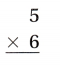5 × 6 = 30.

Explanation:
5 × 6 = 30.

Question 8.10 × 0 = 0.

Explanation:
10 × 0 = (5 × 0) + (5 × 0)
= 0 + 0
= 0.

Question 9.5 × 3 = 15.

Explanation:
5 × 3 = 15.

Problem Solving
Algebra Use the pictures to find the unknown numbers.
Question 10.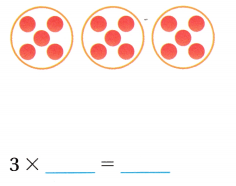3 × 5 = 15.

Explanation:
3 × 5 = 15.

Question 11.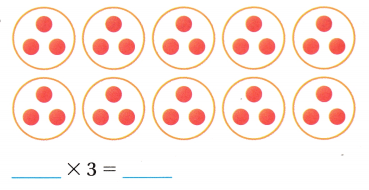10 × 3 = 30.

Explanation:
10 × 3 = (5 × 3) + (5 × 3)
= 30.

Complete the strip diagram to solve.
Question 12.
Marcel played 5 songs on the banjo. If each song lasted 8 minutes, how long did he play?Number of minutes he played = 40.

Explanation:
Number of songs on the banjo Marcel played = 5.
Number of minutes each song lasted = 8.
Number of minutes he played = Number of songs on the banjo Marcel played × Number of minutes each song lasted
= 5 × 8
= 40.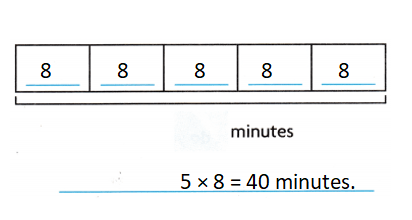Question 13.
There are 6 banjo players. If each player needs 10 sheets of music, how many sheets of music are needed?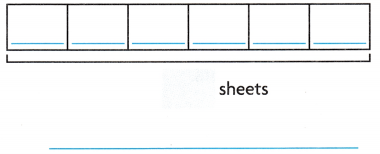Number of sheets of music are needed = 60.

Explanation:
Number of banjo players = 6.
Number of sheets of music each player needs = 10.
Number of sheets of music are needed = Number of banjo players 5 × Number of sheets of music each player needs
= 6 × 10
= (6 × 5) + (6 × 5)
= 30 + 30
= 60.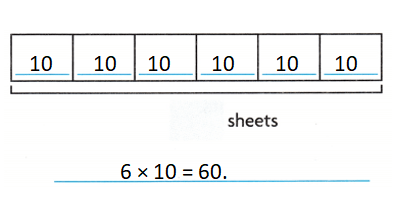Problem Solving
Use the table for 14-16.
Question 14.
Apply John and his dad own 7 banjos. They want to replace the strings on all of them. How many strings should they buy? Write a multiplication sentence to solve.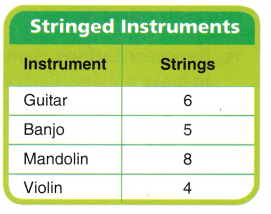Number of strings should they buy = 35.

Explanation:
Number of banjos John and his dad own = 7.
Number of strings banjo has = 5.
Number of strings should they buy = Number of banjos John and his dad own × Number of strings banjo has
= 7 × 5
= 35.

Question 15.
H.O.T. Multi-Step The orchestra has 5 violins and 3 guitars that need new strings. What is the total number of strings that need to be replaced? Explain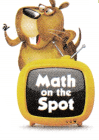Total number of strings that need to be replaced = 38.

Explanation:
Number of violins the orchestra has = 5.
Number of strings violins has = 4.
Number of guitars the orchestra has = 3.
Number of strings guitars has = 6.
Total number of strings that need to be replaced = (Number of violins the orchestra has  × Number of strings violins has) + (Number of guitars the orchestra has × Number of strings guitars has)
= (5 × 4) + (3 × 6)
= 20 + 18
= 38.

Question 16.
Multi-Step Mr. Lemke has 5 guitars, 4 banjos, and 2 mandolins. What is the total number of strings on Mr. Lemke’s instruments? Explain.
Total number of strings on Mr. Lemke’s instruments = 66.

Explanation:
Number of guitars Mr. Lemke has = 5.
Number of strings guitars has = 6.
Number of banjos Mr. Lemke has = 4.
Number of strings banjo has = 5.
Number of mandolins Mr. Lemke has = 2.
Number of strings mandolins has = 8.
Total number of strings on Mr. Lemke’s instruments = (Number of guitars Mr. Lemke has × Number of strings guitars has) + (Number of banjos Mr. Lemke has × Number of strings banjo has) + (Number of mandolins Mr. Lemke has × Number of strings mandolins has)
= (5 × 6) + (4 × 5) + (2 × 8)
= 30 + 20 + 16
= 50 + 16
= 66.

Question 17.
Use Diagrams Mrs. Thompson has 4 shelves with 5 violins on each shelf. How many violins does Mrs. Thompson have? Complete the strip diagram to solve.Number of violins Mrs. Thompson have =20.

Explanation:
Number of shelves Mrs. Thompson has = 4.
Number of violins on each shelf = 5.
Number of violins Mrs. Thompson have = Number of shelves Mrs. Thompson has × Number of violins on each shelf
= 4 × 5
= 20.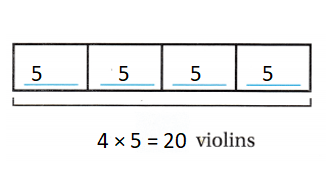Question 18.
H.O.T. Write Math What’s the Error? Mr. James has 3 banjos. Mr. Lewis has 5 times the number of banjos Mr. James has. Riley says Mr. Lewis has 12 banjos. Describe his error.
Riley is not correct saying Mr. Lewis has 12 banjos because number of banjos Mr. Lewis has 15.

Explanation:
Number of banjos Mr. James has = 3.
Mr. Lewis has 5 times the number of banjos Mr. James has.
=> Number of banjos Mr. Lewis has = 5 × Number of banjos Mr. James has
= 5 × 3
= 15.

Question 19.
Representations Sam put together 6 pentominoes to make a shape. Each pentomino has 5 squares. How many squares are in Sam’s shape?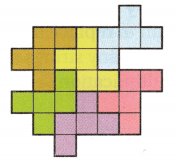(A) 65
(B) 11
(C) 35
(D) 30
Number of squares are in Sam’s shape = 30.
(D) 30.

Explanation:
Number of pentominoes to make a shape Sam put together = 6.
Number of squares each pentomino has = 5.
Number of squares are in Sam’s shape = Number of pentominoes to make a shape Sam put together × Number of squares each pentomino has
= 6 × 5
= 30.

Question 20.
Ali reads for 10 minutes each night before bed. How many minutes does Ali read in 7 nights?
(A) 17 minutes
(B) 70 minutes
(D) 80 minutes
(D) 60 minutes
Number of minutes Ali read in 7 nights = 70.
(B) 70 minutes.

Explanation:
Number of minutes each night before bed Ali reads for = 10.
Number of nights = 7.
Number of minutes Ali read in 7 nights = Number of nights × Number of minutes each night before bed Ali reads for
= 7 × 10
= 70.

Question 21.
Multi-Step Analyze Mark is making 10 kites. He uses 5 yards of ribbon for each kite. He has already made 2 of the kites. How many yards of ribbon will Mark need to make the rest of the kites?
(A) 10 yards
(B) 50 yards
(C) 40 yards
(D) 13 yards
Number of yards of ribbon Mark needs to make the rest of the kites = 40.
(C) 40 yards.

Explanation:
Number of kites Mark is making = 10.
Number of yards of ribbon for each kite he uses = 5.
Number of kites Mark made = 2.
Number of yards of ribbon Mark needs to make the rest of the kites = (Number of kites Mark is making × Number of yards of ribbon for each kite he uses) – (Number of kites Mark made × Number of yards of ribbon for each kite he uses)
= (10 × 5) – (2 × 5)
= 50 – 10
= 40.

Texas Test Prep
Question 22.
There are 5 guitars on each of 5 shelves at a music store. How many guitars are there?
(A) 1o
(B) 20
(C) 15
(D) 25
Number of guitars are there = 25.
(D) 25.

Explanation:
Number of guitars on each shelf at a music store = 5.
Number of shelves at a music store = 5.
Number of guitars are there = Number of guitars on each shelf at a music store × Number of shelves at a music store
= 5 × 5
= 25.

### Texas Go Math Grade 3 Lesson 7.2 Homework and Practice Answer Key

Complete the strip diagram to solve.
Question 1.
A toy store has 6 shelves with 5 game boxes on each shelf. How many game boxes are there?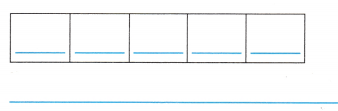Number of game boxes are there = 30.

Explanation:
Number of shelves a toy store has = 6.
Number of game boxes on each shelf = 5.
Number of game boxes are there = Number of shelves a toy store has × Number of game boxes on each shelf
= 6 × 5
= 30.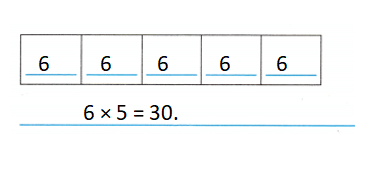Question 2.
A clerk sells 5 boxes of cards. Each box has 10 cards. How many cards are there?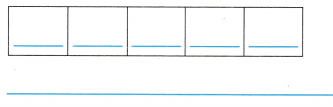Number of cards are there = 50.

Explanation:
Number of boxes of cards a clerk sells = 5.
Number of cards each box has = 10.
Number of cards are there = Number of boxes of cards a clerk sells × Number of cards each box has
= 5 × 10
= 50.Use the table for 3-5.
Question 3.
Jordy buys 5 small ticket books. How many tickets does Jordy have? Write a multiplication sentence to solve.Number of tickets Jordy have = 20.

Explanation:
Number of small ticket books Jordy buys = 5.
Number of tickets of small size = 4.
Number of tickets Jordy have = Number of small ticket books Jordy buys × Number of tickets of small size
= 5 × 4
= 20.

Question 4.
The Dorsey family buys 5 medium books and 1 large book of tickets. What is the total number of tickets the Dorsey family has?
Total number of tickets the Dorsey family has = 33.

Explanation:
Number of medium books of tickets the Dorsey family buys = 5.
Number of tickets of medium size = 5.
Number of large book of tickets the Dorsey family buys = 1.
Number of tickets of large size = 8.
Total number of tickets the Dorsey family has = (Number of medium books of tickets the Dorsey family buys × Number of tickets of medium size) + (Number of large book of tickets the Dorsey family buys × Number of tickets of large size)
= (5 × 5) + (1 × 8)
= 25 + 8
= 33.

Question 5.
Sharon needs 15 tickets to ride the Big Roller Coaster. How many medium books of tickets should she buy?
Number of medium books of tickets should she buy = 3.

Explanation:
Number of tickets to ride the Big Roller Coaster Sharon needs = 15.
Number of tickets of medium size = 5.
Number of medium books of tickets should she buy = Number of tickets to ride the Big Roller Coaster Sharon needs ÷ Number of tickets of medium size
= 15 ÷ 5
= 3.

Problem Solving
Question 6.
Candles come in boxes of 4. Ms. Lee buys 10 boxes of candles for a party. How many candles does Ms. Lee buy?
Number of candles Ms. Lee buys = 40.

Explanation:
Number of Candles in each box = 4.
Number of boxes of candles for a party Ms. Lee buys = 10.
Number of candles Ms. Lee buy = Number of Candles in each box × Number of boxes of candles for a party Ms. Lee buys
= 4 × 10
= 40.

Question 7.
Ms. Lee sets up 9 tables. Each table seats 5 people. How many people can sit at the tables?
Number of people can sit at the tables = 45.

Explanation:
Number of tables Ms. Lee sets up = 9.
Number of people seats on each table = 5.
Number of people can sit at the tables = Number of tables Ms. Lee sets up × Number of people seats on each table
= 9 × 5
= 45.

Texas Test prep
Lesson Check
Question 8.
Five students each borrow 4 books from the library. How many books do the students borrow?
(A) 8
(B) 9
(C) 20
(D) 10
Number of books the students borrowed = 20.
(C) 20.

Explanation:
Number of books from the library each student borrowed = 4.
Number of students = 5.
Number of books the students borrowed = Number of books from the library each student borrowed × Number of students
= 4 × 5
= 20.

Question 9.
There are 10 relay teams on the playground. Each team has 3 students. How many students are on relay teams?
(A) 10
(B) 31
(C) 13
(D) 30
Number of students are on relay teams = 30.
(D) 30.

Explanation:
Number of relay teams on the playground = 10.
Number of students each team has = 3.
Number of students are on relay teams = Number of relay teams on the playground × Number of students each team has
= 10 × 3
= 30.

Question 10.
A grocery store has 8 bags of snack packs on a shelf. Each bag has 5 snack packs. How many snack packs does the store have?
(A) 58
(B) 85
(C) 40
(D) 50
Number of snack packs the store have = 40.
(C) 40.

Explanation:
Number of bags of snack packs on a shelf a grocery store has = 8.
Number of snack packs each bag has = 5.
Number of snack packs the store have = Number of bags of snack packs on a shelf a grocery store has × Number of snack packs each bag has
= 8 × 5
= 40.

Question 11.
Sunrise Goat Farm has 5 pens. Each pen has 9 goats. How many goats are on the farm?
(A) 59
(B) 45
(C) 14
(D) 95
Number of goats are on the farm = 45.
(B) 45.

Explanation:
Number of pens Sunrise Goat Farm has = 5.
Number of goats each pen has = 9.
Number of goats are on the farm = Number of pens Sunrise Goat Farm has × Number of goats each pen has
= 5 × 9
= 45.

Question 12.
Multi-Step Karl is making 9 belts. He uses 5 beads for each belt. He has already made 4 belts. How many beads will Karl need to make the rest of the belts?
(A) 25
(B) 45
(C) 18
(D) 20
Number of beads Karl needs to make the rest of the belts = 25.
(A) 25.

Explanation:
Number of belts Karl is making = 9.
Number of beads for each belt he uses = 5.
Number of beads Karl needs to make the rest of the belts = (Number of belts Karl is making × Number of beads for each belt he uses) – ( Number of belts he has already made  × Number of beads for each belt he uses)
= (9 × 5) – (4 × 5)
= 45 – 20
= 25.

Question 13.
Multi-Step Chris has 5 stacks of DVDs on a shelf. Each stack has 10 DVDs. If Chris adds 2 more identical stacks of DVDs to the shelves, how many DVDs will Chris have?
(A) 20
(B) 50
(C) 17
(D) 70